# Operations on Matrices JEE Notes | EduRev

## JEE : Operations on Matrices JEE Notes | EduRev

The document Operations on Matrices JEE Notes | EduRev is a part of the JEE Course Mathematics (Maths) Class 12.
All you need of JEE at this link: JEE

C. OPERATIONS ON MATRICES

(i) Equality of Matrices : Two matrices A ad B are said to be equal if they are comparable and all the corresponding elements are equal.

Let A = [aij]m × n & B = [bij] p × q. A = B if (i) m = p, n = q (ii) aij = bij for i & j

(ii) Addition of Matrices : Let A and B be two matrices of same order (i.e. comparable matrices). Then A + B is defined to be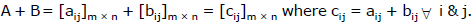(iii)Substraction of Matrices : Let A & B be two matrices of same order. Then A – B is defined as A + (–B) where – B is (–1) B.

(iv)Multiplication of Matrix By Scalar : Let λ be a scalar (real or complex number) & A = [aij]m × n be a matrix. Thus the product λA is defined as λA = [bij]m × n where bij = λaij for all i & j.
Note : If A is a scalar matrix, then A = λI, where λ is the diagonal element.

(v) Properties of Addition & Scalar Multiplication : Consider all matrices of order m × n, whose elements are from a set F (F denote Q, R or C).

Let Mm× n (F) denote the set of all such matrices. Then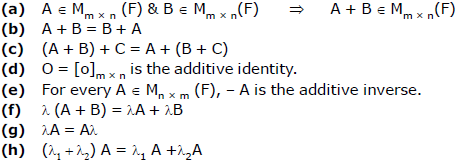Ex.1 For the following pairs of matrices, determine the sum and difference, if they exist.
(a)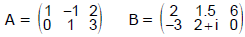(b)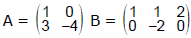Sol. (a) Matrices A and B are 2 × 3 and confirmable for addition and subtraction.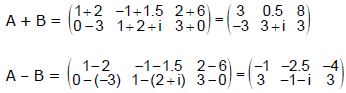(b) Matrix A is 2 × 2, and B is 2 × 3. Since A and B are not the same size, they are not confirmable for addition or subtraction.

Ex.2 Find the additive inverse of the matrix A =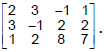Sol. The additive inverse of the 3 × 4 matrix A is the 3 × 4 matrix each of whose elements is the negative of the corresponding element of A. Therefore if we denote the additive inverse of A by – A, we have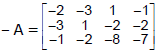. Obviously A + (–A) = (–A) + A = O, where O is the null matrix of the type 3 × 4.

Ex.3 If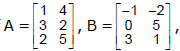find the matrix D such that A + B – D = 0.

Sol.  We have A + B – D = 0  ⇒  (A + B) + (-D) = 0  ⇒  A + B = (-D) = D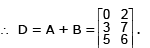Ex.4 If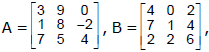verify that 3(A + B) = 3A + 3B.

Sol.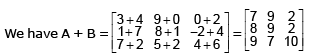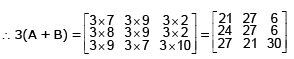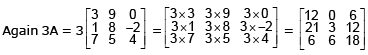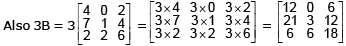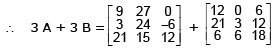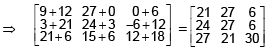∴ 3 (A + B) = 3A + 3B, i.e. the scalar multiplication of matrices distributes over the addition of matrices.

Ex.5 The set of natural numbers N is partitioned into arrays of rows and columns in the form of matrices as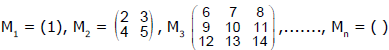and so on. Find the sum of the elements of the diagonal in Mn.

Sol. Let Mn = (aij) where i, j = 1, 2, 3,.........,n.
We first find out a11 for the nth matrix; which is the nth term in the series ;         1, 2, 6,......
Let S = 1 + 2 + 6 + 15 +..... + Tn – 1 + Tn.

Again writing        S = 1 + 2 + 6 +.... + Tn – 1 + Tn

⇒ 0 = 1 + 1 + 4 + 9 +..... + (T– Tn – 1) – Tn ⇒ Tn = 1 + (1 + 4 + 9 +....... upto (n – 1) terms)

= 1 + (1 2 + 22 + 32 + 42 +..... + (n – 1)2)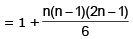(vi) Multiplication of Matrices : Let A and B be two matrices such that the number of columns of A is same as number of rows of B. i.e., A =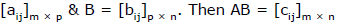where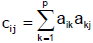which is the dot product of ith row vector of A and jth column vector of B.

Note :
1. The product AB is defined iff the number of columns of A is equal to the number of rows of B. A is called as premultiplier & B is called as post multiplier. AB is defined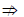BA is defined.
2. In general AB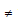BA, even when both the products are defined.
3. A(BC) = (AB) C, whenever it is defined.

(vii) Properties of Matrix Multiplication : Consider all square matrices of order ‘n’. Let Mn (F) denote the set of all square matrices of order n, (where F is Q, R or C). Then
(a) A, B ∈ Mn (F) ⇒ AB ∈ Mn(F)

(b) In general AB ≠ BA

(c) (AB) C = A(BC)

(d) In, the identity matrix of order n, is the multiplicative identity. AIn = A = InA

(e) For every non singular matrix A(i.e., |A| ≠ 0) of Mn (F) there exist a unique (particular) matrix B ∈ Mn (F) so that AB = In = BA. In this case we say that A & B are multiplicative inverse of one another. In notations, we write B = A-1 or A = B-1.

(f) If λ is a scalar (λA) B = λ(AB) = A(λB).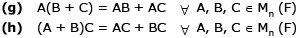Note :

1. Let A = [aij]m × n. Then AIn = A & IA = A, where In & Im are identity matrices of order n & m respectively.

2. For a square matrix A, A2 denotes AA, A3 denotes AAA etc.

Ex.6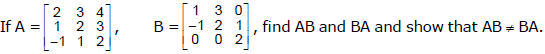Sol.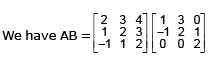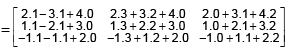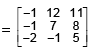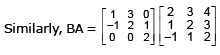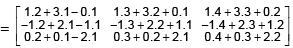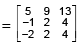The matrix AB is of the type 3 × 3 and the matrix BA is also of the type 3 × 3. But the corresponding elements of these matrices are not equal. Hence AB≠ BA.

Ex.7 Show that for all values of p, q, r, s the matrices,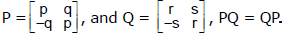Sol.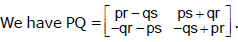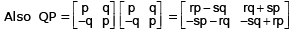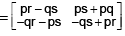for all values of p, q, r,s. Hence PQ = QP, for all values of p, q, r, s.

Ex.8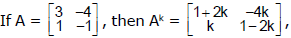where k is any positive integer.

Sol. We shall prove the result by induction on k.

We shall prove the result by induction on k.

We have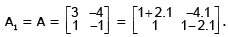Thus the result is true when k = 1.

Now suppose that the result is true for any positive integer k.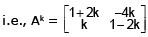where k is any positive integer.

Now we shall show that the result is true for k + 1 if it is true for k. We have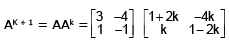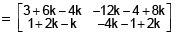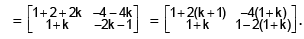Thus the result is true for k + 1 if it is true for k. But it is true for k = 1. Hence by induction it is true for all positive integral value of k.

Ex.9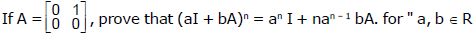where I is the two rowed unit matrix n is a positive integer.

Sol.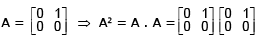= 0  ⇒  A3 = A2 . A = 0  ⇒  A2 = A3 = A4 =...... An = 0

Now by binomial theorem

(a I + b A)n = (a I)n + nC1(a I)n – 1 b A + nC2 (a I)n – 2 (b A)2 +..... + nC(b A)n

= an I + nC1 an – 1 b I A + nCan – 2 b2 I A2 +...... + nCbAn

= an I + n an – 1 b A + 0......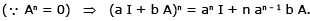Ex.10 If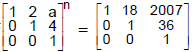then find the value of (n + a).

Sol.  Consider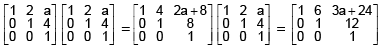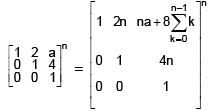Hence n = 9 and 2007 =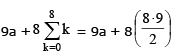⇒ 2007 = 9a + 32 · 9 = 9(a + 32)

⇒   a + 32 = 223  ⇒  a = 191

hence a + n = 200

Ex.11 Find the matrices of transformations T1T2 and T2T1, when T1 is rotation through an angle 60º and T2 is the reflection in the y–axis. Also verify that T1T2T2T1.

Sol.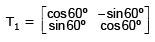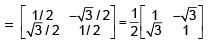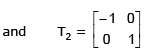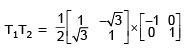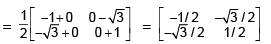...(1)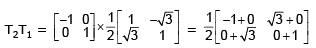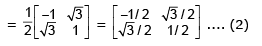It is clear from (1) and (2), T1T2 ≠ T2T1

Ex.12 Find the possible square roots of the two rowed unit matrix I.

Sol.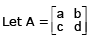be square root of the matrix  =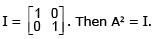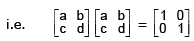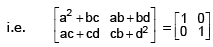Since the above matrices are equal, therefore

a2 + bc =1 ...(i)

ab + bd = 0 ...(ii)

ac + cd = 0 ,,,(iii)

cb + d2 = 0 ....(iv)

must hold simultaneously.
If a + d = 0, the above four equations hold simultaneously if d = –a and a2 + bc =1

Hence one possible square root of I is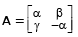where α, β, γ are any three numbers related by the condition α2 + βγ = 1.

If a + d ≠ 0, the above four equations hold simultaneously if b = 0, c = 0, a = 1, d = 1 or if b = 0, c = 0, a = –1, d = –1. Hence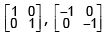i.e.  ± I are other possible square roots of I.

Ex.13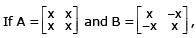then prove that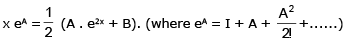Sol.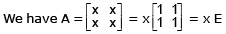...(i)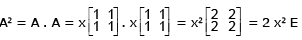...(ii)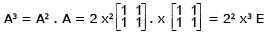...(iii)

Similarly it can be shown that A4 = 23 x4 E, A= 24 x5 E ...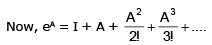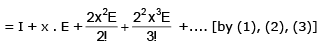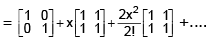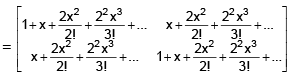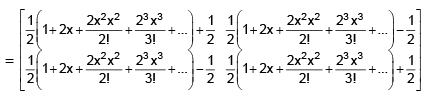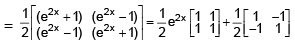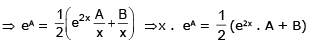D. FURTHER TYPES OF MATRICES

(a) Nilpotent matrix : A square matrix A is said to be nilpotent (of order 2) if, A2 = O.
A square matrix is said to be nilpotent of order p, if p is the least positive integer such that A= O

(b) Idempotent matrix : A square matrix A is said to be idempotent if, A2 = A. eg.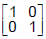is an idempotent matrix.

(c) Involutory matrix : A square matrix A is said to be involutory if A2 = I,I being the identity matrix. eg. A =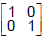is an involutory matrix.

(d) Orthogonal matrix : A square matrix A is said to be an orthogonal matrix if A'A = I = A'A

(e) Unitary matrix : A square matrix A is said to be unitary if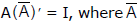is the complex conjugate of A.

Ex.14 Find the number of idempotent diagonal matrices of order n.

Sol. Let A = diag (d1, d2,....., dn) be any diagonal matrix of order n.

now A2 = A . A =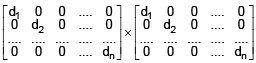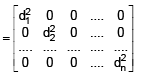But A is idempotent, so A2 = A and hence corresponding elements of A2 and A should be equal

∴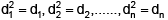or d1 = 0, 1; d2 = 0, 1;.........;dn = 0, 1

⇒ each of d1, d2 ......, dn can be filled by 0 or 1 in two ways.

⇒ Total number of ways of selecting d1, d2,......., dn = 2n

Hence total number of such matrices = 2n.

Ex.15 Show that the matrix A =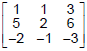is nilpotent and find its index.

Sol.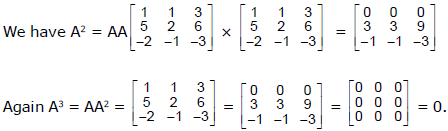Thus 3 is the least positive integer such that A3 = 0. Hence the matrix A is nilpotent of index 3.

Ex.16 If AB = A and BA = B then B'A' = A' and A'B' = B' and hence prove that A' and B' are idempotent.

Sol. We have AB = A ⇒ (AB)' = A' ⇒ B'A' = A'. Also BA = B ⇒ (BA)' = B' ⇒ A'B' = B'.

Now A' is idempotent if A'2 = A'. We have A'2 =A'A' = A' (B'A') = (A'B') A' = B'A' =A'.

∴ A' is idempotent.

Again B'2 = B'B' = B' (A'B') = (B'A') B' = A'B' = B'. ∴ B' is idempotent.

E. TRANSPOSE OF MATRIX

Let A = [aij]m × n. Then the transpose of A is denoted by A'(or AT) and is defined as A' = [bij]n × m where bij = aji  for all i & j

i.e. A' is obtained by rewriting all the rows of A as columns (or by rewriting all the columns of A as rows).

(i) For any matrix A = [aij]m × n, (A')' = A

(ii) Let λ be a scalar & A be a matrix. Then (λA)' = λA'

(iii) (A + B)' = A' + B' & (A - B)' = A' - B' for two comparable matrices A and B.

(iv) (A1 ± A2 ±.... ± An)' = A1' ± A2' ±..... ± An', where Aj are comparable.

(v) Let A = [aij]m × p & B = [bij]p × n, then (AB)' = B'A'

(vi) (A1 A......... An)' = An'. An-1'..................± A2'. A1', provided the product is defined.

(vii) Symmetric & Skew–Symmetric Matrix :  A square matrix A is said to be symmetric if A' = A

i.e. Let A = [aij]n. A is symmetric iff aij = aij for all i & j.
A square matrix A is said to be skew–symmetric if A' = - A

i.e. Let A = [aij]n. A is skew–symmetric iff aij = –aji for all i & j.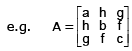is a symmetric matrix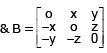is a skew–symmetric matrix.

Note :

1. In skew–symmetric matrix all the diagonal elements are zero. (aij = - aij  ⇒  aij = 0)

2. For any square matrix A, A + A' is symmetric & A -A' is skew - symmetric.

3. Every square matrix can be uniquely expressed as a sum of two square matrices of which one is symmetric and the other is skew–symmetric.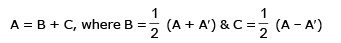Offer running on EduRev: Apply code STAYHOME200 to get INR 200 off on our premium plan EduRev Infinity!

## Mathematics (Maths) Class 12

209 videos|222 docs|124 tests

,

,

,

,

,

,

,

,

,

,

,

,

,

,

,

,

,

,

,

,

,

;